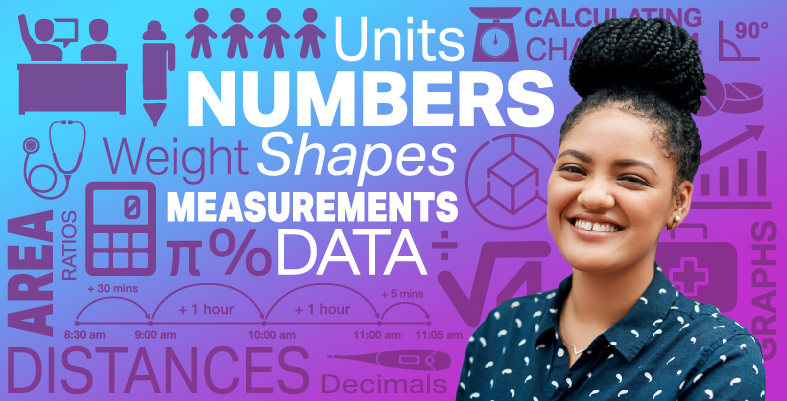Everyday maths for Health and Social Care and Education Support 2

Start this free course now. Just create an account and sign in. Enrol and complete the course for a free statement of participation or digital badge if available.

Free course

# 1.1 Converting units of measure in the same system

Imagine you are doing some baking with a group of children and go to the wholesale shop to buy flour. You need 200 g of flour for each batch of cookies you will be making and need to make 30 batches. You can buy a 5 kg bag of flour but aren’t sure if that will be enough.

In order to work out if you have enough flour, you need both measurements to be in the same unit – kg or g – before you can do the calculation. For units of measure that are in the same system (so you are not dealing with converting cm and inches for example) it’s a simple process to convert one measurement into another. In most cases you will just need to multiply or divide by 10, 100 or 1,000. Take a look at the diagrams below which explain how to convert each type of measurement unit.

## Length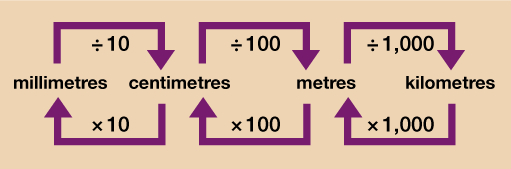Figure 1 A conversion chart for length

## Weight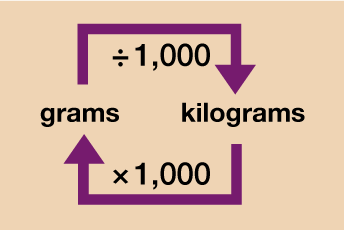Figure 2 A conversion chart for weight

## Money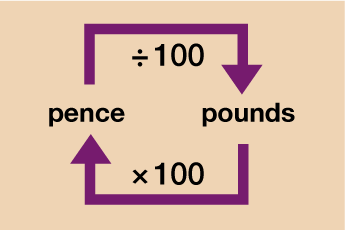Figure 3 A conversion chart for money

## Capacity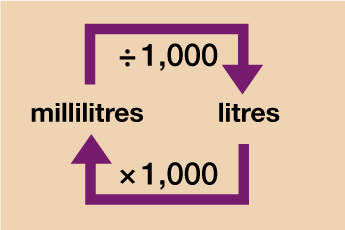Figure 4 A conversion chart for capacity

You’ll learn how to convert between different currencies and different units of measure (kilograms (kg) to pounds (lb) for example) later in this section. For now, have a go at the activity below and test your conversion skills along with your problem solving ones!

## Activity 2: Solving conversion problems

1. A teenage boy is 1.6 m tall. Over the next year, they have a growth spurt and grow a further 12 cm.

How tall is the boy at the end of the year?

2. A baby is born weighing 3.6 kg. In its first week of life it loses 250g.

How much does the baby weigh at the end of the week?

3. A water cooler comes with water containers that hold 12 l of water. The cups provided for use hold 150 ml. You estimate that in the clinic waiting room there are an average of 25 cups of water drunk each day.

How many 12 l bottles will you need for one week (7 days). Round your answer to the nearest whole number.

4. As a general rule, a baby should drink 150 ml of milk for each 1 kg of their body weight in a 24 hour period. Baby Max weighs 4.2 kg. You buy a tub of formula milk that says it will make 4 litres of milk.

If Max drinks the recommended amount each day, will the tub of formula last for a full week (7 days)?

1. 1 m = 100 cm so 1.6 × 100 = 160 cm

160 cm + 12 cm = 172 cm or 1.72 m

2. 1 kg = 1,000 g so 3.6 × 1,000 = 3,600g

3,600 – 250 = 3350 g or 3.35 kg

3. 1 litre = 1,000 ml so 12 l = 12 × 1,000 = 12,000 ml

Each day 25 cups are drunk 25 × 150 ml = 3,750 ml a day

A working week is 7 days:

• 3,750 × 7 = 26,250 ml for the week

• 26,250 ÷ 12,000 = 2.1875

Rounded to the nearest whole number this is 2 containers per week.

4. 1 litre = 1,000 ml so 4 litres = 4 × 1,000 = 4,000 ml of formula milk that can be made

4.2 kg × 150 ml = 630 ml in a 24 hour period

630 ml × 7 days = 4,410 ml in a full week

No, there is not enough formula to make all the milk Max will need over a week.

Hopefully you will now be feeling confident with converting units of measure within the same system. You’ll need to know how to do this and be able to remember how to convert from one to another by multiplying or dividing.

If you are being asked to convert between litres and gallons or US dollars and British pounds however, you won’t be expected to know the conversion rate, you’ll be given it in the question. The next section will show you how to use these conversion rates and you’ll be able to practise solving problems that require you to do this.

## Summary

In this section you have learned:

• that different units are used for measurement

• unit selection depends on the item or object being measured

• how to convert between units in the same system.

FSM_SSH_2